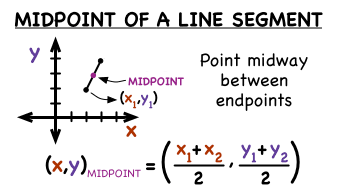# How do you find the midpoint of the line segment joining (5,2) (3,8)?

May 28, 2018

The midpoint is at $\left(4 , 5\right)$.

#### Explanation:

The midpoint formula is shown here:We are given the two endpoints, so we can plug it into the formula to find the midpoint. Notice that the formula is same as the average of the two x-values and y-values.

$\text{Midpoint} = \left(\frac{5 + 3}{2} , \frac{2 + 8}{2}\right)$

$\quad \quad \quad \quad \quad \quad \quad \quad = \left(\frac{8}{2} , \frac{10}{2}\right)$

$\quad \quad \quad \quad \quad \quad \quad \quad = \left(4 , 5\right)$

Hope this helps!